# 矩阵连乘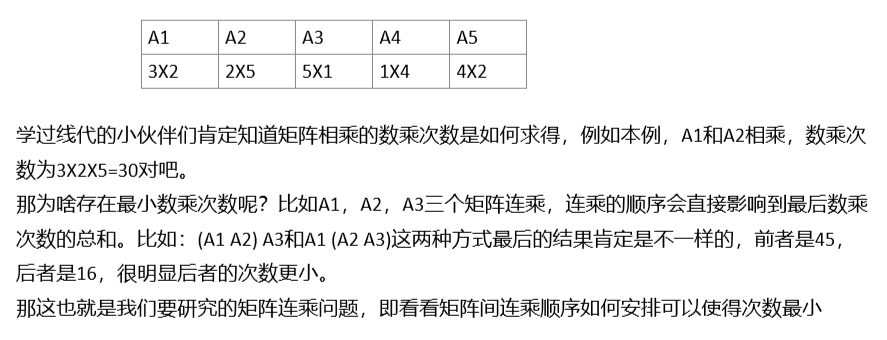OK，那既然用动态规划来做，那么依旧是五步走：

1、确定dp数组的含义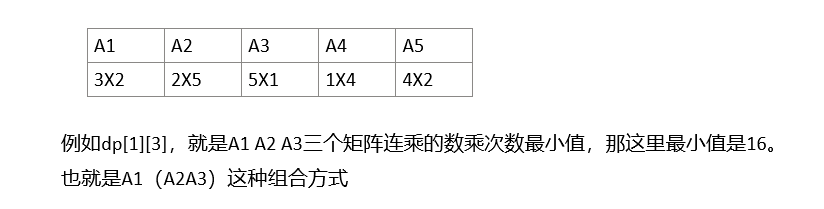2、确定递推公式，这个稍后做解释

3、dp数组的初始化，这个稍后做解释

4、确定遍历dp数组的顺序

5、遍历（更新）dp数组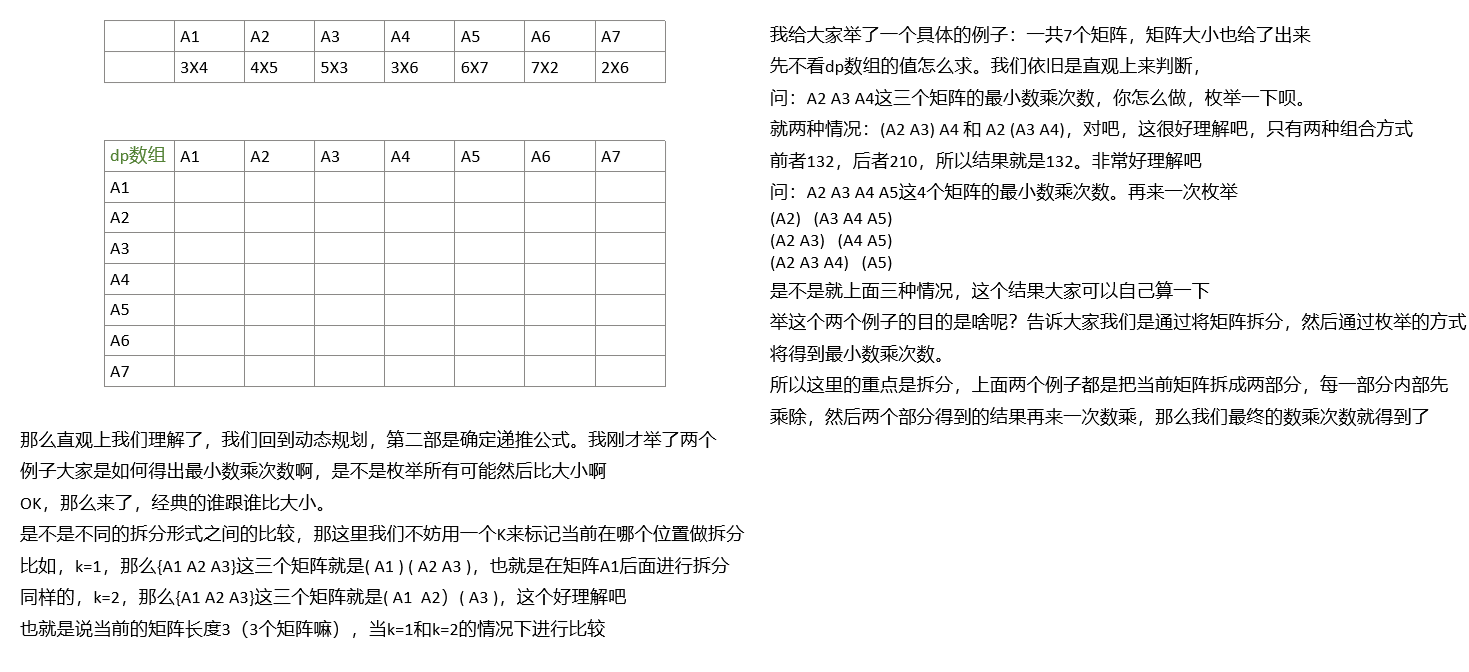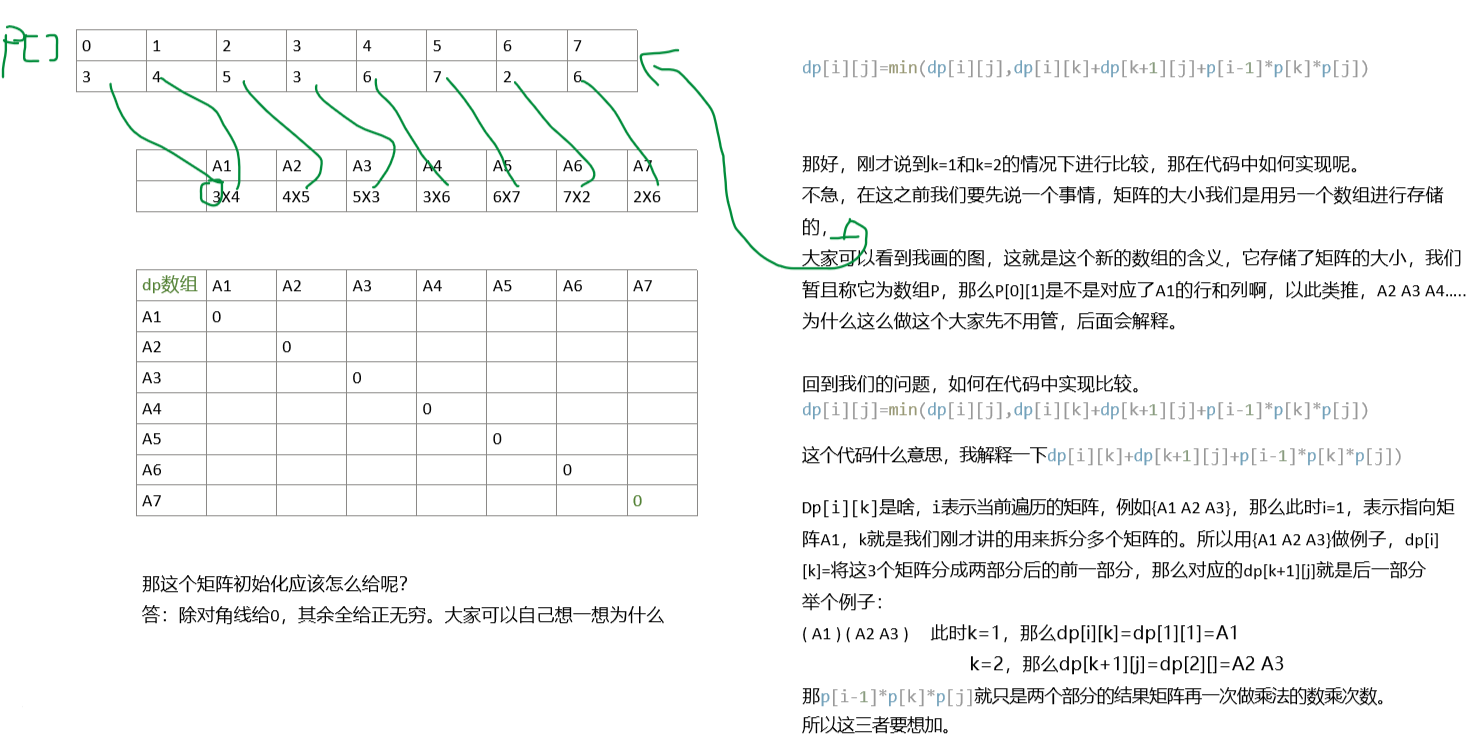``````vector<int> p={3,4,5,3,6,7,2,6};
int n=p.size()-1;

vector<vector<int>> dp(p.size(),vector<int>(p.size(),INT32_MAX));

void func()
{
for(int d=2;d<=n;d++)
{
for(int i=1;i<=n-d+1;i++)
{
int j=i+d-1;
dp[i][j]=INT32_MAX;
for(int k=i;k<j;k++)
dp[i][j]=min(dp[i][j],dp[i][k]+dp[k+1][j]+p[i-1]*p[k]*p[j]);
}
}
cout<<dp[p.size()-1]<<endl;
}``````# 石子合并

​ 石子合并其实和矩阵连乘的逻辑差不多的，区别就在状态转移方程上，整体的实现方式是和矩阵连乘很相似的，大家看完就会发现，无论是dp二维数组还是最终的输出都是和矩阵连乘类似的。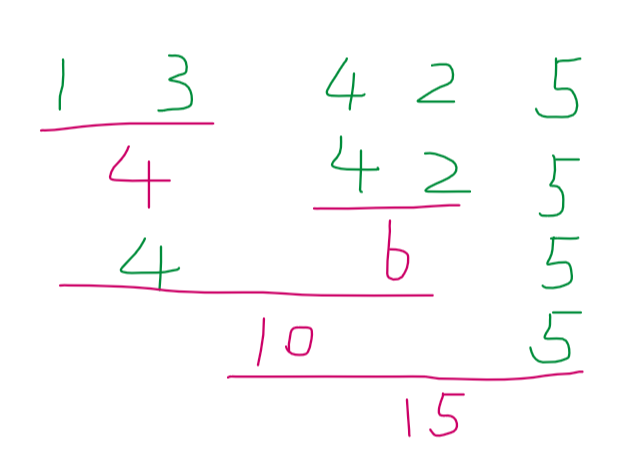1、确定dp二维数组中元素的含义

2、确定状态转移方程，这个稍后解释

3、确定dp数组的初始化，这个稍后解释

4、确定遍历的顺序，需要几重循环，每个循环分别代表什么意思

5、更新（遍历）dp数组，将值填入dp数组。这个不解释了啊，这么多动态规划的题讲下来，这个步骤是干啥的你要在不知道，多少有点伤可爱的心了。

``````for(int len=2;len<=a.size();len++)
{
for(int i=1;i+len-1<=a.size();i++)
{
int j=i+len-1;
for(int k=i;k<j;k++)
{
//真的只有这个状态转移方程不一样
dp[i][j]=min(dp[i][j],dp[i][k]+dp[k+1][j]+s[j]-s[i-1]);
}
}
}``````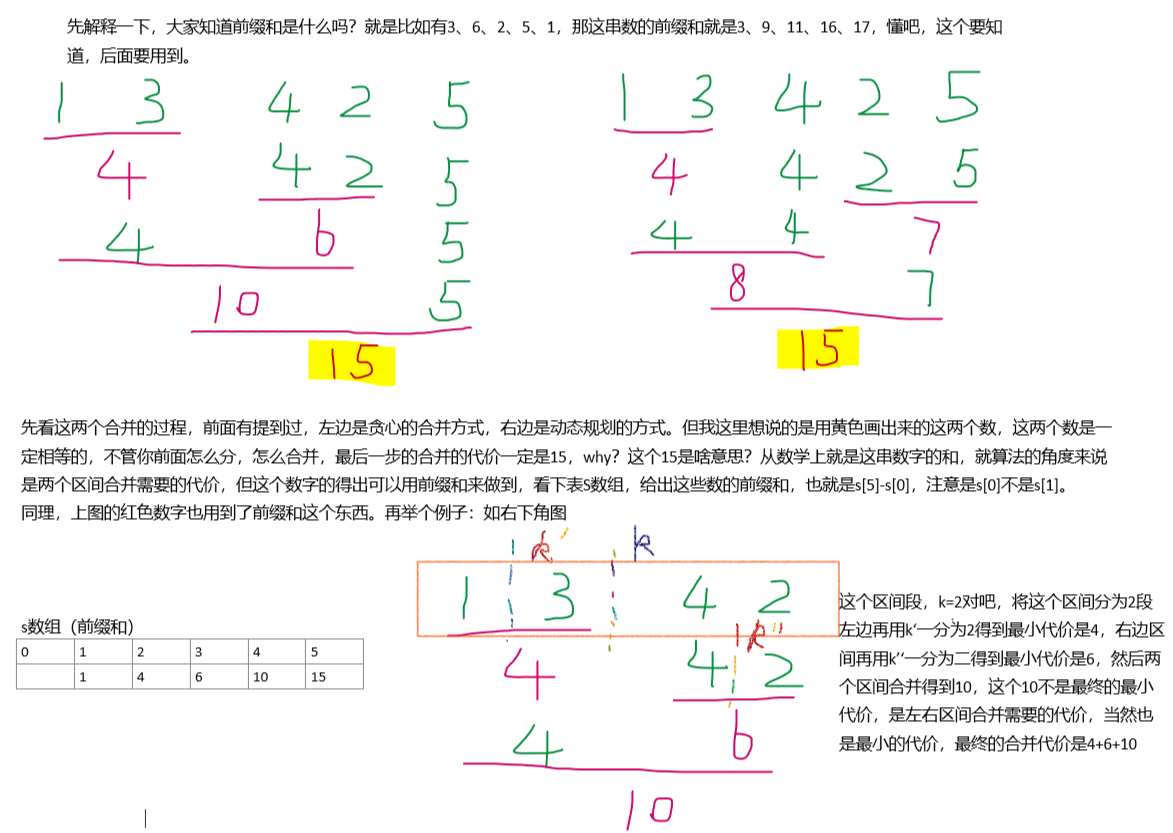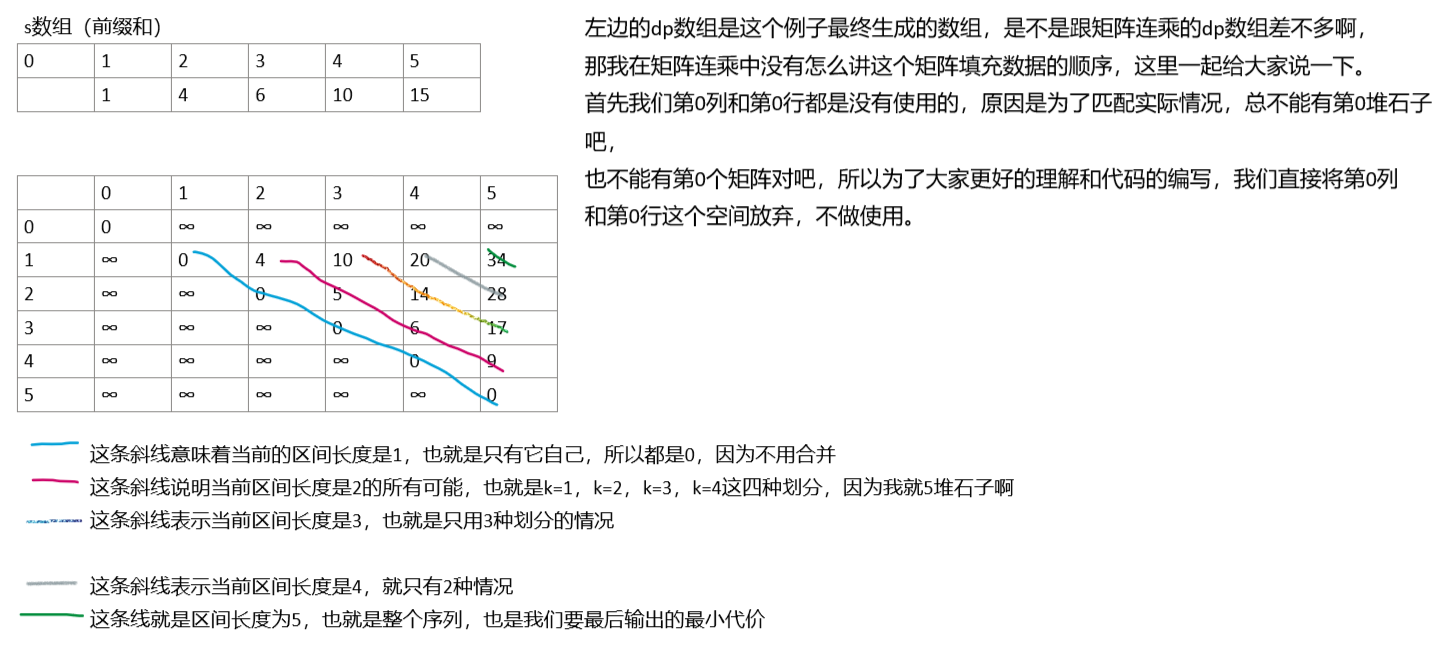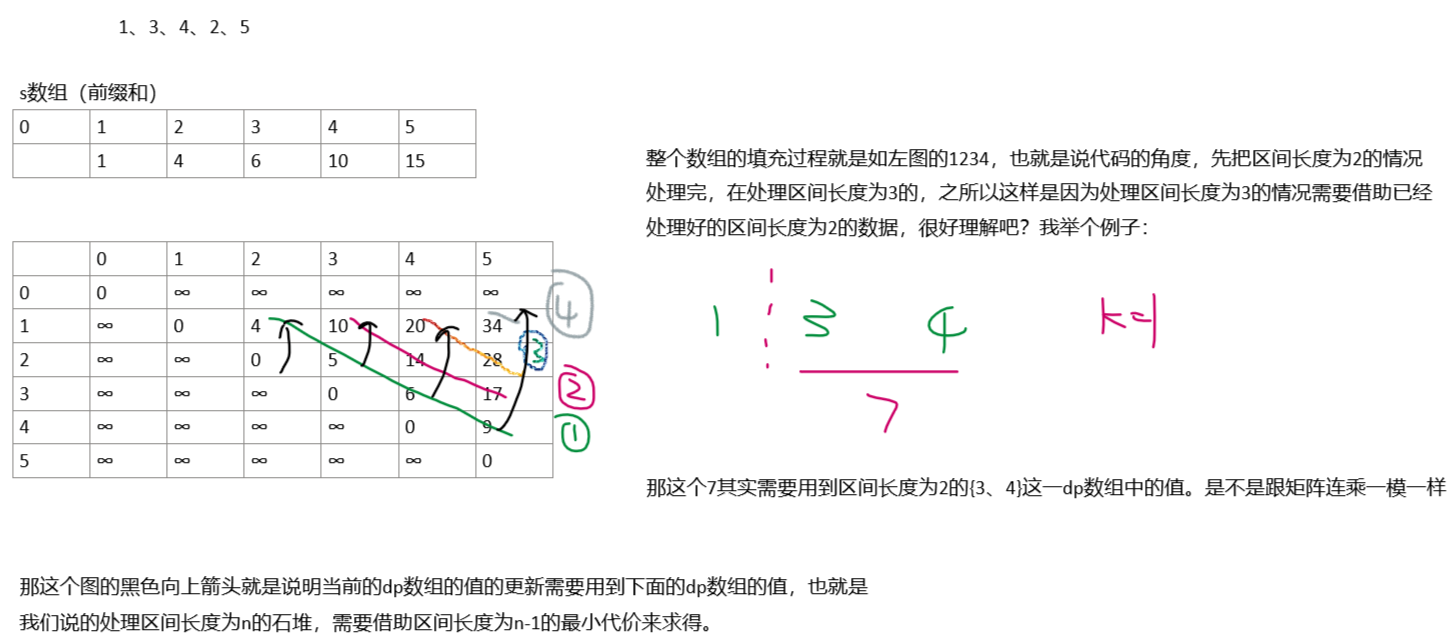``````#include<iostream>
#include<vector>
#include<iomanip>
using namespace std;

#define N 5

vector<int> a={0,1,3,2,4,5};
vector<int> s(a.size()+1);
vector<vector<int>> dp(a.size()+1,vector<int>(a.size()+1,INT32_MAX));

void func()
{
for(int len=2;len<=a.size();len++)
{
for(int i=1;i+len-1<=a.size();i++)
{
int j=i+len-1;
for(int k=i;k<j;k++)
{
dp[i][j]=min(dp[i][j],dp[i][k]+dp[k+1][j]+s[j]-s[i-1]);
}
}
}
cout<<dp[N-1]<<endl;
}

int main()
{
for(int i=1;i<a.size();i++)
{
s[i]=s[i-1]+a[i];
dp[i][i]=0;
}

func();

for(int i=0;i<a.size();i++)
{
for(int j=0;j<a.size();j++)
cout<<setw(10)<<dp[i][j]<<" ";
cout<<endl;
}
return 0;
}``````

lionの金库